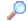Search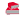Directory

Desktop Functions:

Smart Device Functions: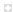Glossary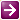Show Recent ChangesSubscribe (RSS)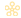Misc. Pages
getkeyboardstate (user32)

Summary

#### C# Signature:

[DllImport("user32.dll", SetLastError = true)]
[return: MarshalAs(UnmanagedType.Bool)]
static extern bool GetKeyboardState(byte [] lpKeyState);

#### VB Signature:

<DllImport("user32.dll", SetLastError := true)> _
Private Shared Function GetKeyboardState(ByVal keyState() As Byte) As Boolean
End Function

'or...
Private Declare Function GetKeyboardState Lib "user32" (ByVal keyState() As Byte) As Boolean

#### Notes:

In some cases this function will always return the same array, independent of actual keyboard state. This is due to Windows not updating the virtual key array internally. It has been found that declaring and calling GetKeyState on any key before calling GetKeyboardState will solve this issue.

#### C#:

using System;
using System.Runtime.InteropServices;
using System.ComponentModel;

namespace PInvokeSample {
public static class Keyboard {

public static int GetKeyState(){
byte[] keys = new byte;

//Get pressed keys
if(!NativeMethods.GetKeyboardState(keys)){
int err = Marshal.GetLastWin32Error();
throw new Win32Exception(err);
}

for(int i = 0; i < 256; i++){

byte key = keys[i];

//Logical 'and' so we can drop the low-order bit for toggled keys, else that key will appear with the value 1!
if((key & 0x80) != 0){

//This is just for a short demo, you may want this to return
//multiple keys!
return (int)key;
}
}
return -1;
}
}
}

using System;
using System.Runtime.InteropServices;

namespace PInvokeSample {
internal static class NativeMethods {

[DllImport("User32.dll")]
[return: MarshalAs(UnmanagedType.Bool)]
internal static extern bool GetKeyboardState(byte[] lpKeyState);

}
}

#### VB:

Imports System.Runtime.InteropServices

Public Class Form1

Private Sub Button1_Click(ByVal sender As System.Object, ByVal e As System.EventArgs) Handles Button1.Click
Me.Text = "Caps:" & NativeMethods.CapsLockState.ToString
Me.Text &= ", Num: " & NativeMethods.NumLockState.ToString
Me.Text &= ", Scroll: " & NativeMethods.ScrollLockState.ToString
End Sub

End Class

Public Class NativeMethods

Private Shared keyState() As Byte

<DllImport("user32.dll")> _
Private Shared Function GetKeyboardState(ByVal keyState() As Byte) As Boolean
End Function

Private Shared Sub Update()
keyState = New Byte(256) {}
Dim result As Boolean = GetKeyboardState(keyState)
' Check for error:
If result = False Then
Debug.WriteLine("GetKeyBoardState error: " & Marshal.GetLastWin32Error)
Throw New Exception("GetKeyBoardState error: " & Marshal.GetLastWin32Error)
End If
End Sub

Public Enum LightState
Off
[On]
End Enum

' Example - the keyboard lights...

Public Shared ReadOnly Property CapsLockState() As LightState
Get
Update()
Dim isOn As Boolean = (keyState(Keys.CapsLock) = 1)
Return IIf(isOn, LightState.On, LightState.Off)
End Get
End Property

Public Shared ReadOnly Property NumLockState() As LightState
Get
Update()
Dim isOn As Boolean = (keyState(Keys.NumLock) = 1)
Return IIf(isOn, LightState.On, LightState.Off)
End Get
End Property

Public Shared ReadOnly Property ScrollLockState() As LightState
Get
Update()
Dim isOn As Boolean = (keyState(Keys.Scroll) = 1)
Return IIf(isOn, LightState.On, LightState.Off)
End Get
End Property

End Class

#### Alternative Managed API:

using System;
using System.Collections.Generic;
using System.Runtime.InteropServices;
using System.Text;

namespace PInvoke.Net
{
public class Keyboard
{
[DllImport( "user32.dll" )]
static extern short GetKeyState( VirtualKeyStates nVirtKey );

public static bool IsKeyPressed( VirtualKeyStates testKey )
{
bool keyPressed = false;
short result = GetKeyState( testKey );

switch( result )
{
case 0:
// Not pressed and not toggled on.
keyPressed = false;
break;

case 1:
// Not pressed, but toggled on
keyPressed = false;
break;

default:
// Pressed (and may be toggled on)
keyPressed = true;
break;
}

return keyPressed;
}

public enum VirtualKeyStates : int
{
VK_LBUTTON = 0x01,
VK_RBUTTON = 0x02,
VK_CANCEL = 0x03,
VK_MBUTTON = 0x04,
//
VK_XBUTTON1 = 0x05,
VK_XBUTTON2 = 0x06,
//
VK_BACK = 0x08,
VK_TAB = 0x09,
//
VK_CLEAR = 0x0C,
VK_RETURN = 0x0D,
//
VK_SHIFT = 0x10,
VK_CONTROL = 0x11,
VK_PAUSE = 0x13,
VK_CAPITAL = 0x14,
//
VK_KANA = 0x15,
VK_HANGEUL = 0x15, /* old name - should be here for compatibility */
VK_HANGUL = 0x15,
VK_JUNJA = 0x17,
VK_FINAL = 0x18,
VK_HANJA = 0x19,
VK_KANJI = 0x19,
//
VK_ESCAPE = 0x1B,
//
VK_CONVERT = 0x1C,
VK_NONCONVERT = 0x1D,
VK_ACCEPT = 0x1E,
VK_MODECHANGE = 0x1F,
//
VK_SPACE = 0x20,
VK_PRIOR = 0x21,
VK_NEXT = 0x22,
VK_END = 0x23,
VK_HOME = 0x24,
VK_LEFT = 0x25,
VK_UP = 0x26,
VK_RIGHT = 0x27,
VK_DOWN = 0x28,
VK_SELECT = 0x29,
VK_PRINT = 0x2A,
VK_EXECUTE = 0x2B,
VK_SNAPSHOT = 0x2C,
VK_INSERT = 0x2D,
VK_DELETE = 0x2E,
VK_HELP = 0x2F,
//
VK_LWIN = 0x5B,
VK_RWIN = 0x5C,
VK_APPS = 0x5D,
//
VK_SLEEP = 0x5F,
//
VK_MULTIPLY = 0x6A,
VK_SEPARATOR = 0x6C,
VK_SUBTRACT = 0x6D,
VK_DECIMAL = 0x6E,
VK_DIVIDE = 0x6F,
VK_F1 = 0x70,
VK_F2 = 0x71,
VK_F3 = 0x72,
VK_F4 = 0x73,
VK_F5 = 0x74,
VK_F6 = 0x75,
VK_F7 = 0x76,
VK_F8 = 0x77,
VK_F9 = 0x78,
VK_F10 = 0x79,
VK_F11 = 0x7A,
VK_F12 = 0x7B,
VK_F13 = 0x7C,
VK_F14 = 0x7D,
VK_F15 = 0x7E,
VK_F16 = 0x7F,
VK_F17 = 0x80,
VK_F18 = 0x81,
VK_F19 = 0x82,
VK_F20 = 0x83,
VK_F21 = 0x84,
VK_F22 = 0x85,
VK_F23 = 0x86,
VK_F24 = 0x87,
//
VK_NUMLOCK = 0x90,
VK_SCROLL = 0x91,
//
VK_OEM_NEC_EQUAL = 0x92, // '=' key on numpad
//
VK_OEM_FJ_JISHO = 0x92, // 'Dictionary' key
VK_OEM_FJ_MASSHOU = 0x93, // 'Unregister word' key
VK_OEM_FJ_TOUROKU = 0x94, // 'Register word' key
VK_OEM_FJ_LOYA = 0x95, // 'Left OYAYUBI' key
VK_OEM_FJ_ROYA = 0x96, // 'Right OYAYUBI' key
//
VK_LSHIFT = 0xA0,
VK_RSHIFT = 0xA1,
VK_LCONTROL = 0xA2,
VK_RCONTROL = 0xA3,
//
VK_BROWSER_BACK = 0xA6,
VK_BROWSER_FORWARD = 0xA7,
VK_BROWSER_REFRESH = 0xA8,
VK_BROWSER_STOP = 0xA9,
VK_BROWSER_SEARCH = 0xAA,
VK_BROWSER_FAVORITES = 0xAB,
VK_BROWSER_HOME = 0xAC,
//
VK_VOLUME_DOWN = 0xAE,
VK_VOLUME_UP = 0xAF,
VK_MEDIA_NEXT_TRACK = 0xB0,
VK_MEDIA_PREV_TRACK = 0xB1,
VK_MEDIA_STOP = 0xB2,
VK_MEDIA_PLAY_PAUSE = 0xB3,
VK_LAUNCH_MAIL = 0xB4,
VK_LAUNCH_MEDIA_SELECT = 0xB5,
VK_LAUNCH_APP1 = 0xB6,
VK_LAUNCH_APP2 = 0xB7,
//
VK_OEM_1 = 0xBA, // ';:' for US
VK_OEM_PLUS = 0xBB, // '+' any country
VK_OEM_COMMA = 0xBC, // ',' any country
VK_OEM_MINUS = 0xBD, // '-' any country
VK_OEM_PERIOD = 0xBE, // '.' any country
VK_OEM_2 = 0xBF, // '/?' for US
VK_OEM_3 = 0xC0, // '`~' for US
//
VK_OEM_4 = 0xDB, // '[{' for US
VK_OEM_5 = 0xDC, // '\|' for US
VK_OEM_6 = 0xDD, // ']}' for US
VK_OEM_7 = 0xDE, // ''"' for US
VK_OEM_8 = 0xDF,
//
VK_OEM_AX = 0xE1, // 'AX' key on Japanese AX kbd
VK_OEM_102 = 0xE2, // "<>" or "\|" on RT 102-key kbd.
VK_ICO_HELP = 0xE3, // Help key on ICO
VK_ICO_00 = 0xE4, // 00 key on ICO
//
VK_PROCESSKEY = 0xE5,
//
VK_ICO_CLEAR = 0xE6,
//
VK_PACKET = 0xE7,
//
VK_OEM_RESET = 0xE9,
VK_OEM_JUMP = 0xEA,
VK_OEM_PA1 = 0xEB,
VK_OEM_PA2 = 0xEC,
VK_OEM_PA3 = 0xED,
VK_OEM_WSCTRL = 0xEE,
VK_OEM_CUSEL = 0xEF,
VK_OEM_ATTN = 0xF0,
VK_OEM_FINISH = 0xF1,
VK_OEM_COPY = 0xF2,
VK_OEM_AUTO = 0xF3,
VK_OEM_ENLW = 0xF4,
VK_OEM_BACKTAB = 0xF5,
//
VK_ATTN = 0xF6,
VK_CRSEL = 0xF7,
VK_EXSEL = 0xF8,
VK_EREOF = 0xF9,
VK_PLAY = 0xFA,
VK_ZOOM = 0xFB,
VK_NONAME = 0xFC,
VK_PA1 = 0xFD,
VK_OEM_CLEAR = 0xFE
}
}
}

Documentation

Do you have...

• helpful tips or sample code to share for using this API in managed code?
• corrections to the existing content?
• variations of the signature you want to share?
• additional languages you want to include?

Select "Edit This Page" on the right hand toolbar and edit it! Or add new pages containing supporting types needed for this API (structures, delegates, and more).Access PInvoke.net directly from VS:Terms of UseEdit This PageFind ReferencesShow Printable Version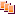Revisions Show changes